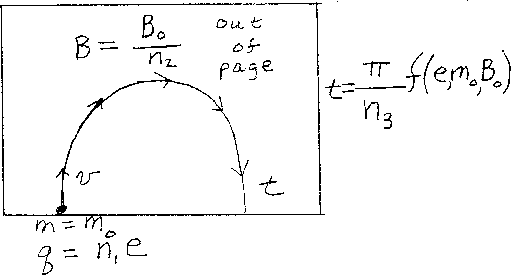Problem C4: The figure above shows a mass spectrometer. There is a uniform magnetic field B which points out of the screen. A molecule, with a charge of n1e, enters the region with a speed v. The molecule has a mass of m0. After traveling in a semi circle, the molecule hits the same side of the spectrometer that it entered. If the magnetic field has a strength of B=B0/n2, what is the time t that the molecule travels before it hits the side? If the time t is equal to t = π f(e,m0,B0)/n3? What is n3? Here, the function f(e,m0,B0) is the combination of these three values in the metric system that give units of time in seconds.n1 = n2 = Input n3:
If you are currently in my class, you can record your grade by entering your name and student ID number (without the leading zeros) below and clicking on "record grade".
 First Name = Last Name = ID = Problem: